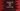# static in typescript with example## static in TypeScript and how to use:

Starting from ES6, typescript supports static properties. static members can be accessed without creating an object of a class. We just need the class name and member name to access it using a dot notation.

``<Class>.<Static>``

We can have any method or any field of a class as static. static keyword is used to mark one value as static.

In this post, we will learn how to use static with different examples.

### Example of static method and property in TypeScript:

Let’s take a look at the below example:

``````class Util{
static MULTIPLIER: number = 13;

static getResult(num: number){
return num * this.MULTIPLIER;
}
}

console.log(Util.MULTIPLIER);
console.log(Util.getResult(5));``````

For this example,

• MULTIPLIER is a static variable in the Util class.
• getResult is a static method that takes one number as a parameter and returns one value by multiplying that number with MULTIPLIER.
• We are accessing both of these static members using Util.

If we create an object of Util and try to access its members like below :

``````let util = new Util();
console.log(util.MULTIPLIER);``````

### Accessing static values from non-static method:

In the above example, we are accessing static value from a static method. Let’s see how can we access static values from a non-static method:

``````class Util{
static MULTIPLIER: number = 13;

getResult(num: number){
return num * Util.MULTIPLIER;
}
}

let util = new Util();
console.log(util.getResult(5));``````

Here,

• We have one getResult function that is not static and one number MULTIPLIER that is static.
• From the non-static method getResult, we are accessing the static value MULTIPLIER.
• For that, we are using Util. instead of this.

It will run.

### Two members with the same name:

We can have two members, one with static and another with non static with the same name. For example,

``````class Util{
static MULTIPLIER: number = 13;
MULTIPLIER: number = 14;
}

let util = new Util();
console.log(util.MULTIPLIER);
console.log(Util.MULTIPLIER);``````

It will give different results for both class level and object level.

It will print:

``````14
13``````